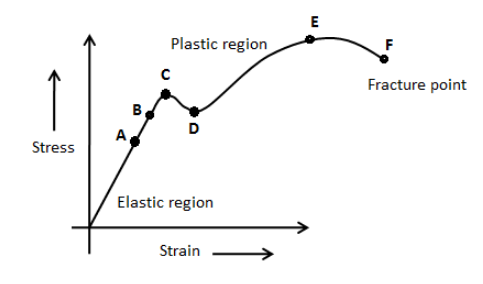State Hooke’s law, with graphical representation.

305.7k+ views

Hint:The applied force per unit area is known as stress. The compression or elongation of the material in response to the stress is known as strain. Every material undergoes strain in response to the stress. Hooke’s law defines the dependence of stress on strain.
We know that when we apply a force to a deforming body, it either undergoes compression or elongation. The applied force per unit area is known as stress and it is denoted by $\sigma$. The elongation or compression of the material in response to the stress depends on the property of the material.
Hooke’s law of elasticity states that the elongation or compression of the material is proportional to the stress within its elastic limit. We know that the compression or elongation of the material in response to the stress is known as strain. Therefore, we can state Hooke’s law as the strain is directly proportional to the stress within the elastic limit. We can express Hooke’s law as,
$F = – kx$
Here, k is the force constant and x is the elongation or compression in the material.
We know that if the material regains its original dimensions after the stress is removed, the property is known as elasticity and if the material does not regain its original dimensions after the stress is removed, the property is known as plasticity.
The following graph shows the region of elasticity and plasticity according to Hooke’s law.In the above figure, the strain is directly proportional to the stress up to the point C. We call this region an elastic region. After the point C, we can observe that the stress is not proportional to the strain. We call this region a plastic region. With increasing stress, we come across a point F where the stress causes breaking of the material.
Note:Students often could not differentiate between spring force and Hooke’s law. Both forces have the same expression but the spring force has a constant known as spring constant and in Hooke’s law, the constant k represents force constant. Note that Hooke’s law is valid only in the elastic region.

We know that when we apply a force to a deforming body, it either undergoes compression or elongation. The applied force per unit area is known as stress and it is denoted by $\sigma$. The elongation or compression of the material in response to the stress depends on the property of the material.

Hooke’s law of elasticity states that the elongation or compression of the material is proportional to the stress within its elastic limit. We know that the compression or elongation of the material in response to the stress is known as strain. Therefore, we can state Hooke’s law as the strain is directly proportional to the stress within the elastic limit. We can express Hooke’s law as,

$F = – kx$

Here, k is the force constant and x is the elongation or compression in the material.

We know that if the material regains its original dimensions after the stress is removed, the property is known as elasticity and if the material does not regain its original dimensions after the stress is removed, the property is known as plasticity.

The following graph shows the region of elasticity and plasticity according to Hooke’s law.In the above figure, the strain is directly proportional to the stress up to the point C. We call this region an elastic region. After the point C, we can observe that the stress is not proportional to the strain. We call this region a plastic region. With increasing stress, we come across a point F where the stress causes breaking of the material.

Note:Students often could not differentiate between spring force and Hooke’s law. Both forces have the same expression but the spring force has a constant known as spring constant and in Hooke’s law, the constant k represents force constant. Note that Hooke’s law is valid only in the elastic region.

Last updated date: 01st Sep 2023

Total views: 305.7k

Views today: 6.05k

Recently Updated Pages

10 Examples of Evaporation in Daily Life with Explanations10 Examples of Friction in Our Daily LifeWhat do you mean by public facilitiesDifference between hardware and softwareTrending doubts

How do you solve x2 11x + 28 0 using the quadratic class 10 maths CBSEFill the blanks with the suitable prepositions 1 The class 9 english CBSEHow many kilometers is the earth from the sun class 8 physics CBSEDifference between Prokaryotic cell and Eukaryotic class 11 biology CBSEDifference Between Plant Cell and Animal CellThe equation xxx + 2 is satisfied when x is equal to class 10 maths CBSEGive 10 examples for herbs , shrubs , climbers , creepersDifferentiate between homogeneous and heterogeneous class 12 chemistry CBSE10 examples of law on inertia in our daily lifeYou are watching: State Hooke’s law, with graphical representation.. Info created by GBee English Center selection and synthesis along with other related topics.